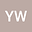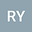••The paper is concerned with the following Schr\”{o}dinger-Poisson system $$\left\{\begin{array}{ll} -\Delta u+u+\phi u=|u|^{p-1}u+|u|^4u, &x\in \mathbb{R}^3, \\[0.25cm] -\Delta\phi=u^2, &x\in \mathbb{R}^3, \end{array} \right.$$ where \$3